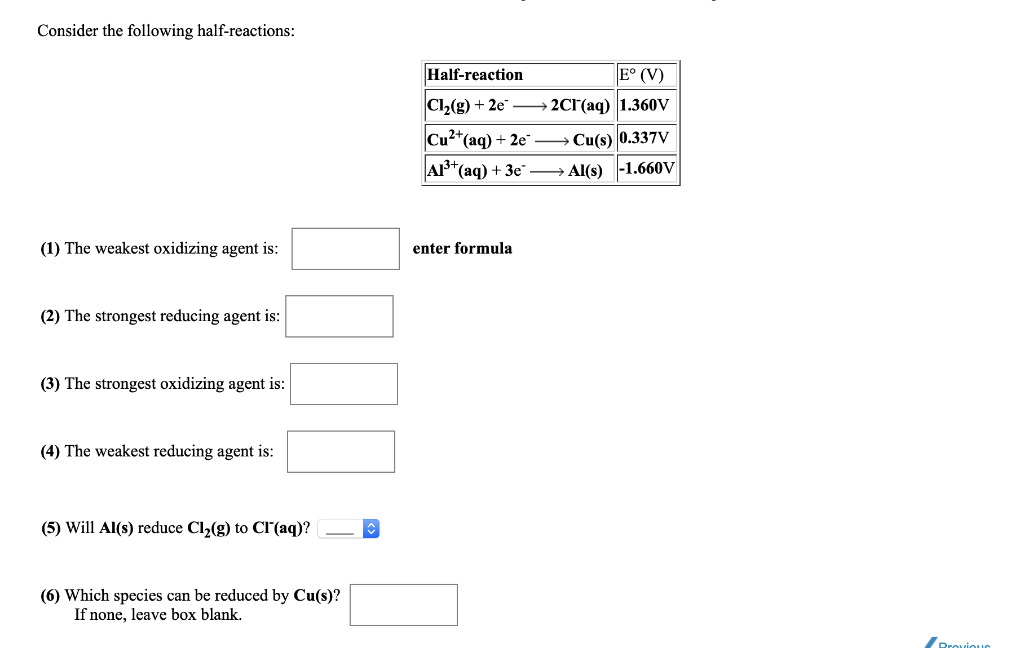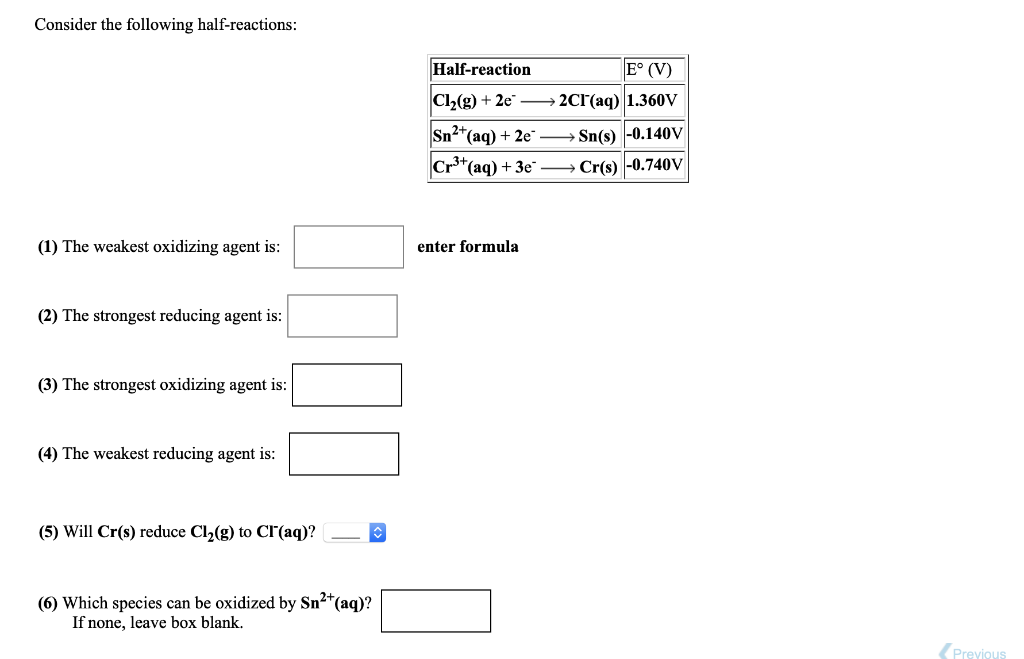# Question Solved1 Answer5 Consider the following half-reactions: Half-reaction E° (V) Cl2(g) + 2e - →2Cl(aq) 1.360V Cu2+(aq) + 2e Cu(s) 0.337V A1+ (aq) + 3e" —— Al(s) -1.660V (1) The weakest oxidizing agent is: enter formula (2) The strongest reducing agent is: (3) The strongest oxidizing agent is: (4) The weakest reducing agent is: (5) Will Al(s) reduce Cl2(g) to Cl(aq)? () Which species can be reduced by Cu(s)? If none, leave box blank. Consider the following half-reactions: Half-reaction E° (V) Cl2(g) + 2e - →2Cl(aq) 1.360V Sn2+ (aq) + 2e Cr3+ (aq) + 3e Sn(s) -0.140V Cr(s) -0.740V (1) The weakest oxidizing agent is: enter formula (2) The strongest reducing agent is: (3) The strongest oxidizing agent is: (4) The weakest reducing agent is: (5) Will Cr(s) reduce Cl2(g) to Cl(aq)? (6) Which species can be oxidized by Sn2+(aq)? If none, leave box blank. Previous5Transcribed Image Text: Consider the following half-reactions: Half-reaction E° (V) Cl2(g) + 2e - →2Cl(aq) 1.360V Cu2+(aq) + 2e Cu(s) 0.337V A1+ (aq) + 3e" —— Al(s) -1.660V (1) The weakest oxidizing agent is: enter formula (2) The strongest reducing agent is: (3) The strongest oxidizing agent is: (4) The weakest reducing agent is: (5) Will Al(s) reduce Cl2(g) to Cl(aq)? () Which species can be reduced by Cu(s)? If none, leave box blank. Consider the following half-reactions: Half-reaction E° (V) Cl2(g) + 2e - →2Cl(aq) 1.360V Sn2+ (aq) + 2e Cr3+ (aq) + 3e Sn(s) -0.140V Cr(s) -0.740V (1) The weakest oxidizing agent is: enter formula (2) The strongest reducing agent is: (3) The strongest oxidizing agent is: (4) The weakest reducing agent is: (5) Will Cr(s) reduce Cl2(g) to Cl(aq)? (6) Which species can be oxidized by Sn2+(aq)? If none, leave box blank. Previous
More
Transcribed Image Text: Consider the following half-reactions: Half-reaction E° (V) Cl2(g) + 2e - →2Cl(aq) 1.360V Cu2+(aq) + 2e Cu(s) 0.337V A1+ (aq) + 3e" —— Al(s) -1.660V (1) The weakest oxidizing agent is: enter formula (2) The strongest reducing agent is: (3) The strongest oxidizing agent is: (4) The weakest reducing agent is: (5) Will Al(s) reduce Cl2(g) to Cl(aq)? () Which species can be reduced by Cu(s)? If none, leave box blank. Consider the following half-reactions: Half-reaction E° (V) Cl2(g) + 2e - →2Cl(aq) 1.360V Sn2+ (aq) + 2e Cr3+ (aq) + 3e Sn(s) -0.140V Cr(s) -0.740V (1) The weakest oxidizing agent is: enter formula (2) The strongest reducing agent is: (3) The strongest oxidizing agent is: (4) The weakest reducing agent is: (5) Will Cr(s) reduce Cl2(g) to Cl(aq)? (6) Which species can be oxidized by Sn2+(aq)? If none, leave box blank. Previous